## Inferring Causes and Effects from Daily Data

Doing different activities we often are interesting how they impact each other. For example, if we visit different links on Internet, we might want to know how this action impacts our motivation for doing some specific things. In other words we are interesting in inferring importance of causes for effects from our daily activities data.

In this post we will look at few ways to detect relationships between actions and results using machine learning algorithms and python.

Our data example will be artificial dataset consisting of 2 columns: URL and Y.
URL is our action and we want to know how it impacts on Y. URL can be link0, link1, link2 wich means links visited, and Y can be 0 or 1, 0 means we did not got motivated, and 1 means we got motivated.

The first thing we do hot-encoding link0, link1, link3 in 0,1 and we will get 3 columns as below.Sample of data after one hot encoding

So we have now 3 features, each for each URL. Here is the code how to do hot-encoding to prepare our data for cause and effect analysis.

filename = "C:\\Users\\drm\\data.csv"
dataframe = pandas.read_csv(filename)

dataframe=pandas.get_dummies(dataframe)
cols = dataframe.columns.tolist()
cols.insert(len(dataframe.columns)-1, cols.pop(cols.index('Y')))
dataframe = dataframe.reindex(columns= cols)

print (len(dataframe.columns))

#output
#4



Now we can apply feature extraction algorithm. It allows us select features according to the k highest scores.

# feature extraction
test = SelectKBest(score_func=chi2, k="all")
fit = test.fit(X, Y)
# summarize scores
numpy.set_printoptions(precision=3)
print ("scores:")
print(fit.scores_)

for i in range (len(fit.scores_)):
print ( str(dataframe.columns.values[i]) + "    " + str(fit.scores_[i]))
features = fit.transform(X)

print (list(dataframe))

numpy.set_printoptions(threshold=numpy.inf)

scores:
[11.475  0.142 15.527]
URL_link0    11.475409836065575
URL_link1    0.14227166276346598
URL_link2    15.526957539965377
['URL_link0', 'URL_link1', 'URL_link2', 'Y']

Another algorithm that we can use is <strong>ExtraTreesClassifier</strong> from python machine learning library sklearn.

from sklearn.ensemble import ExtraTreesClassifier
from sklearn.feature_selection import SelectFromModel

clf = ExtraTreesClassifier()
clf = clf.fit(X, Y)
print (clf.feature_importances_)
model = SelectFromModel(clf, prefit=True)
X_new = model.transform(X)
print (X_new.shape)

#output
#[0.424 0.041 0.536]
#(150, 2)



The above two machine learning algorithms helped us to estimate the importance of our features (or actions) for our Y variable. In both cases URL_link2 got highest score.

There exist other methods. I would love to hear what methods do you use and for what datasets and/or problems. Also feel free to provide feedback or comments or any questions.

Below is python full source code

# -*- coding: utf-8 -*-
import pandas
import numpy
from sklearn.feature_selection import SelectKBest
from sklearn.feature_selection import chi2

filename = "C:\\Users\\drm\\data.csv"
dataframe = pandas.read_csv(filename)

dataframe=pandas.get_dummies(dataframe)
cols = dataframe.columns.tolist()
cols.insert(len(dataframe.columns)-1, cols.pop(cols.index('Y')))
dataframe = dataframe.reindex(columns= cols)

print (dataframe)
print (len(dataframe.columns))

array = dataframe.values
X = array[:,0:len(dataframe.columns)-1]
Y = array[:,len(dataframe.columns)-1]
print ("--X----")
print (X)
print ("--Y----")
print (Y)

# feature extraction
test = SelectKBest(score_func=chi2, k="all")
fit = test.fit(X, Y)
# summarize scores
numpy.set_printoptions(precision=3)
print ("scores:")
print(fit.scores_)

for i in range (len(fit.scores_)):
print ( str(dataframe.columns.values[i]) + "    " + str(fit.scores_[i]))
features = fit.transform(X)

print (list(dataframe))

numpy.set_printoptions(threshold=numpy.inf)
print ("features")
print(features)

from sklearn.ensemble import ExtraTreesClassifier
from sklearn.feature_selection import SelectFromModel

clf = ExtraTreesClassifier()
clf = clf.fit(X, Y)
print ("feature_importances")
print (clf.feature_importances_)
model = SelectFromModel(clf, prefit=True)
X_new = model.transform(X)
print (X_new.shape)


## Prediction on Next Stock Market Correction

On Feb. 6, 2018, the stock market officially entered “correction” territory. A stock market correction is defined as a drop of at least 10% or more for an index or stock from its recent high.  During one week the stock data prices (closed price) were decreasing for many stocks. Are there any signals that can be used to predict next stock market correction?

I pulled historical data from 20 stocks selected randomly and then created python program that counts how many stocks (closed price) were decreased, increased or did not change for each day (comparing with previous day). The numbers then converted into percentage. So if all 20 stock closed prices decreased at some day it would be 100%. For now I was just looking at % of decreased stocks per day. Below is the graph for decreasing stocks. Highlighted zone A is when we many decreasing stocks during the correction.Number of decreasing stocks per day in %

### Observations

I did not find good strong signal to predict market correction but probably more analysis needed. However before this correction there was some increasing trend for number of stocks that close at lower prices. This is shown below. On this graph the trend line can be viewed as indicator of stock market direction.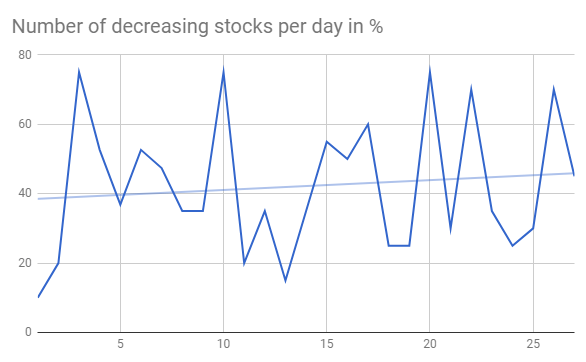Number of decreasing stocks per day before correction in %

### Python Source Code to download Stock Data

Here is the script that was used to download data:

from pandas_datareader import data as pdr
import time

# put below actual symbols as many as you need
symbols=['XXX','XXX', 'XXX', ...... 'XXX']

def get_data (symbol):

data = pdr.get_data_google(symbol,'1970-01-01','2018-02-19')
path="C:\\Users\\stocks\\"
data.to_csv( path + symbol+".csv")

return data

for symbol in symbols:
get_data(symbol)
time.sleep(7)


### Script for Stock Data Analysis

Here is the program that takes downloaded data and counts the number of decreased/increased/same stocks per day. The results are saved in the file and also plotted. Plots are shown after source code below.

And here is the link to the data output from the below program.

# -*- coding: utf-8 -*-

import os

path="C:\\Users\\stocks\\"
from datetime import datetime
import pandas as pd
import numpy as np

def days_between(d1, d2):
d1 = datetime.strptime(d1, "%Y-%m-%d")
d2 = datetime.strptime(d2, "%Y-%m-%d")
print (d1)
print (d2)
return abs((d2 - d1).days)

i=10000   # index to replace date
j=20      # index for stock symbols
k=5       # other attributes
data = np.zeros((i,j,k))
symbols=[]

count=0

# get index of previous trade day
# because there is no trades on weekend or holidays
# need to calculate prvious trade day index instead
# of just subracting 1
def get_previous_ind(row_ind, col_count ):

k=1
print (str(row_ind) + "   " + str(col_count))
while True:
if  data[row_ind-k][col_count] == 1:
return row_ind-k
else:
k=k+1

if k > 1000 :
print ("ERROR: PREVIOUS ROW IS NOT FOUND")
return -1

dates=["" for i in range(10000) ]
# read the entries
listOfEntries = os.scandir(path)
for entry in  listOfEntries:

if entry.is_file():
print(entry.name)
stock_data = pd.read_csv (str(path) + str(entry.name))
symbols.append (entry.name)

for index, row in stock_data.iterrows():
ind=days_between(row['Date'], "2002-01-01")

dates[ind] = row['Date']
data[ind][count] = 1
data[ind][count] = row['Close']

if (index > 1):
print(entry.name)
prev_ind=get_previous_ind(ind, count)
delta= 1000*(row['Close'] - data[prev_ind][count])
change=0
if (delta > 0) :
change = 1
if (delta < 0) :
change = -1
data[ind][count] = change
data[ind][count] = 1

count=count+1

upchange=[0 for i in range(10000)]
downchange=[0 for i in range(10000)]
zerochange=[0 for i in range(10000)]
datesnew = ["" for i in range(10000) ]
icount=0
for i in range(10000):
total=0
for j in range (count):

if data[i][j] == 1 :
datesnew[icount]=dates[i]
total=total+1
if (data[i][j] ==0):
zerochange[icount]=zerochange[icount]+1
if (data[i][j] ==1):
upchange[icount]=upchange[icount] + 1
if (data[i][j] == - 1):
downchange[icount]=downchange[icount] + 1

if (total != 0) :
upchange[icount]=100* upchange[icount] / total
downchange[icount]=100* downchange[icount] / total
zerochange[icount]=100* zerochange[icount] / total
print (str(upchange[icount]) + "  " +  str(downchange[icount]) + "  " + str(zerochange[icount]))
icount=icount+1

df=pd.DataFrame({'Date':datesnew, 'upchange':upchange, 'downchange':downchange, 'zerochange':zerochange })
print (df)
df.to_csv("changes.csv", encoding='utf-8', index=False)

import matplotlib.pyplot as plt

downchange=downchange[icount-200:icount]
upchange=upchange[icount-200:icount]
zerochange=zerochange[icount-200:icount]

# Two subplots, the axes array is 1-d
f, axarr = plt.subplots(3, sharex=True)
axarr.plot(downchange)
axarr.set_title('downchange')
axarr.plot(upchange)
axarr.set_title('upchange')
axarr.plot(zerochange)
axarr.set_title('zerochange')
plt.show()Number of stocks increasing decreasing same in %

## How to Create Data Visualization for Association Rules in Data Mining

Association rule learning is used in machine learning for discovering interesting relations between variables. Apriori algorithm is a popular algorithm for association rules mining and extracting frequent itemsets with applications in association rule learning. It has been designed to operate on databases containing transactions, such as purchases by customers of a store (market basket analysis).  Besides market basket analysis this algorithm can be applied to other problems. For example in web user navigation domain we can search for rules like customer who visited web page A and page B also visited page C.

Python sklearn library does not have Apriori algorithm but recently I come across post  where python library MLxtend was used for Market Basket Analysis. MLxtend has modules for different tasks. In this post I will share how to create data visualization for association rules in data mining using MLxtend for getting association rules and NetworkX module for charting the diagram. First we need to get association rules.

### Getting Association Rules from Array Data

To get association rules you can run the following code

dataset = [['Milk', 'Onion', 'Nutmeg', 'Kidney Beans', 'Eggs', 'Yogurt'],
['Dill', 'Onion', 'Nutmeg', 'Kidney Beans', 'Eggs', 'Yogurt'],
['Milk', 'Apple', 'Kidney Beans', 'Eggs'],
['Milk', 'Unicorn', 'Corn', 'Kidney Beans', 'Yogurt'],
['Corn', 'Onion', 'Onion', 'Kidney Beans', 'Ice cream', 'Eggs']]

import pandas as pd
from mlxtend.preprocessing import OnehotTransactions
from mlxtend.frequent_patterns import apriori

oht = OnehotTransactions()
oht_ary = oht.fit(dataset).transform(dataset)
df = pd.DataFrame(oht_ary, columns=oht.columns_)
print (df)

frequent_itemsets = apriori(df, min_support=0.6, use_colnames=True)
print (frequent_itemsets)

association_rules(frequent_itemsets, metric="confidence", min_threshold=0.7)
rules = association_rules(frequent_itemsets, metric="lift", min_threshold=1.2)
print (rules)

"""
Below is the output
support                     itemsets
0       0.8                       [Eggs]
1       1.0               [Kidney Beans]
2       0.6                       [Milk]
3       0.6                      [Onion]
4       0.6                     [Yogurt]
5       0.8         [Eggs, Kidney Beans]
6       0.6                [Eggs, Onion]
7       0.6         [Kidney Beans, Milk]
8       0.6        [Kidney Beans, Onion]
9       0.6       [Kidney Beans, Yogurt]
10      0.6  [Eggs, Kidney Beans, Onion]

antecedants            consequents  support  confidence  lift
0  (Kidney Beans, Onion)                 (Eggs)      0.6        1.00  1.25
1   (Kidney Beans, Eggs)                (Onion)      0.8        0.75  1.25
2                (Onion)   (Kidney Beans, Eggs)      0.6        1.00  1.25
3                 (Eggs)  (Kidney Beans, Onion)      0.8        0.75  1.25
4                (Onion)                 (Eggs)      0.6        1.00  1.25
5                 (Eggs)                (Onion)      0.8        0.75  1.25

"""


### Confidence and Support in Data Mining

To select interesting rules we can use best-known constraints which are a minimum thresholds on confidence and support.
Support is an indication of how frequently the itemset appears in the dataset.
Confidence is an indication of how often the rule has been found to be true. 

support=rules.as_matrix(columns=['support'])
confidence=rules.as_matrix(columns=['confidence'])


Below is the scatter plot for support and confidence:Association rules – scatter plot

And here is the python code to build scatter plot. Since few points here have the same values I added small random values to show all points.

import random
import matplotlib.pyplot as plt

for i in range (len(support)):
support[i] = support[i] + 0.0025 * (random.randint(1,10) - 5)
confidence[i] = confidence[i] + 0.0025 * (random.randint(1,10) - 5)

plt.scatter(support, confidence,   alpha=0.5, marker="*")
plt.xlabel('support')
plt.ylabel('confidence')
plt.show()


### How to Create Data Visualization with NetworkX for Association Rules in Data Mining

To represent association rules as diagram, NetworkX python library is utilized in this post. Here is the association rule example :
(Kidney Beans, Onion) ==> (Eggs)

Directed graph below is built for this rule and shown below. Arrows are drawn as just thicker blue stubs. The node with R0 identifies one rule, and it will have always incoming and outcoming edges. Incoming edge(s) will represent antecedants and the stub (arrow) will be next to node.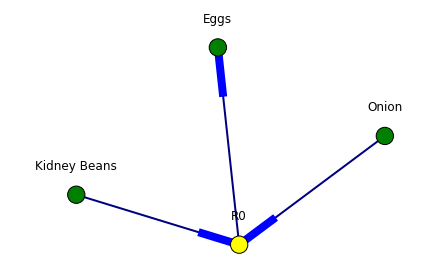Below is the example of graph for all rules extracted from example dataset.Here is the source code to build association rules with NetworkX. To call function use draw_graph(rules, 6)

def draw_graph(rules, rules_to_show):
import networkx as nx
G1 = nx.DiGraph()

color_map=[]
N = 50
colors = np.random.rand(N)
strs=['R0', 'R1', 'R2', 'R3', 'R4', 'R5', 'R6', 'R7', 'R8', 'R9', 'R10', 'R11']

for i in range (rules_to_show):
G1.add_nodes_from(["R"+str(i)])

for a in rules.iloc[i]['antecedants']:

G1.add_nodes_from([a])

G1.add_edge(a, "R"+str(i), color=colors[i] , weight = 2)

for c in rules.iloc[i]['consequents']:

G1.add_nodes_from()

G1.add_edge("R"+str(i), c, color=colors[i],  weight=2)

for node in G1:
found_a_string = False
for item in strs:
if node==item:
found_a_string = True
if found_a_string:
color_map.append('yellow')
else:
color_map.append('green')

edges = G1.edges()
colors = [G1[u][v]['color'] for u,v in edges]
weights = [G1[u][v]['weight'] for u,v in edges]

pos = nx.spring_layout(G1, k=16, scale=1)
nx.draw(G1, pos, edges=edges, node_color = color_map, edge_color=colors, width=weights, font_size=16, with_labels=False)

for p in pos:  # raise text positions
pos[p] += 0.07
nx.draw_networkx_labels(G1, pos)
plt.show()


### Data Visualization for Online Retail Data Set

To get real feeling and testing on visualization we can take available online retail store dataset and apply the code for association rules graph. For downloading retail data and formatting some columns the code from  was used.

Below are the result of scatter plot for support and confidence. To build the scatter plot seaborn library was used this time. Also you can find below visualization for association rules (first 10 rules) for retail data set.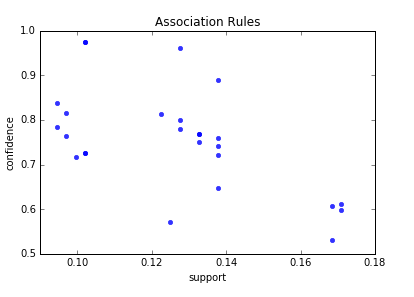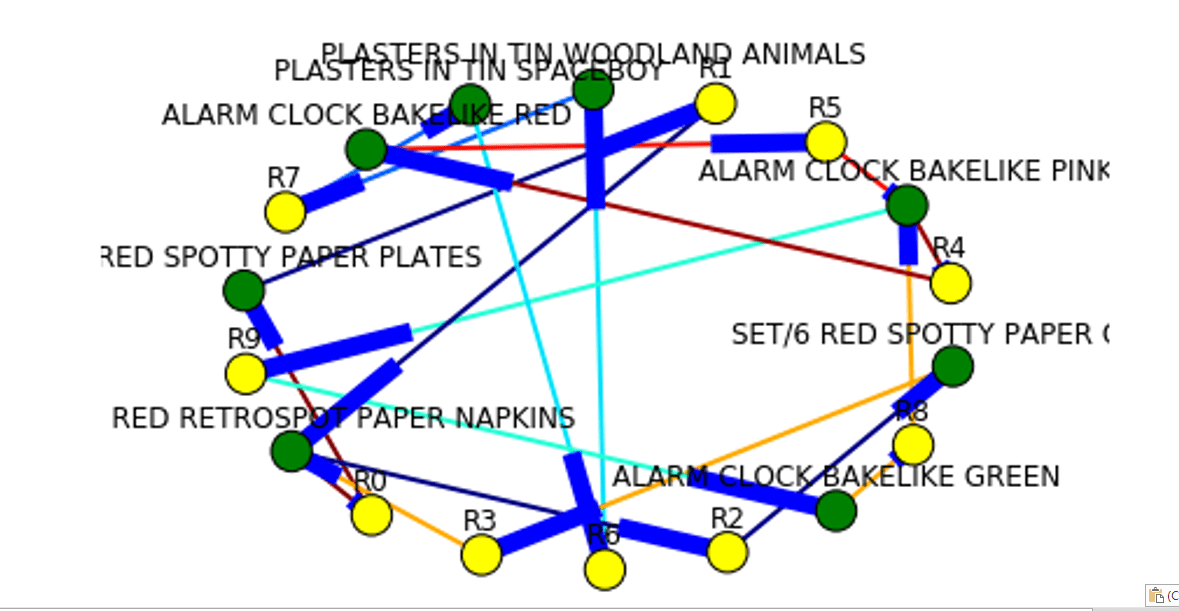Here is the python full source code for data visualization association rules in data mining.



dataset = [['Milk', 'Onion', 'Nutmeg', 'Kidney Beans', 'Eggs', 'Yogurt'],
['Dill', 'Onion', 'Nutmeg', 'Kidney Beans', 'Eggs', 'Yogurt'],
['Milk', 'Apple', 'Kidney Beans', 'Eggs'],
['Milk', 'Unicorn', 'Corn', 'Kidney Beans', 'Yogurt'],
['Corn', 'Onion', 'Onion', 'Kidney Beans', 'Ice cream', 'Eggs']]

import pandas as pd
from mlxtend.preprocessing import OnehotTransactions
from mlxtend.frequent_patterns import apriori

oht = OnehotTransactions()
oht_ary = oht.fit(dataset).transform(dataset)
df = pd.DataFrame(oht_ary, columns=oht.columns_)
print (df)

frequent_itemsets = apriori(df, min_support=0.6, use_colnames=True)
print (frequent_itemsets)

from mlxtend.frequent_patterns import association_rules

association_rules(frequent_itemsets, metric="confidence", min_threshold=0.7)
rules = association_rules(frequent_itemsets, metric="lift", min_threshold=1.2)
print (rules)

support=rules.as_matrix(columns=['support'])
confidence=rules.as_matrix(columns=['confidence'])

import random
import matplotlib.pyplot as plt

for i in range (len(support)):
support[i] = support[i] + 0.0025 * (random.randint(1,10) - 5)
confidence[i] = confidence[i] + 0.0025 * (random.randint(1,10) - 5)

plt.scatter(support, confidence,   alpha=0.5, marker="*")
plt.xlabel('support')
plt.ylabel('confidence')
plt.show()

import numpy as np

def draw_graph(rules, rules_to_show):
import networkx as nx
G1 = nx.DiGraph()

color_map=[]
N = 50
colors = np.random.rand(N)
strs=['R0', 'R1', 'R2', 'R3', 'R4', 'R5', 'R6', 'R7', 'R8', 'R9', 'R10', 'R11']

for i in range (rules_to_show):
G1.add_nodes_from(["R"+str(i)])

for a in rules.iloc[i]['antecedants']:

G1.add_nodes_from([a])

G1.add_edge(a, "R"+str(i), color=colors[i] , weight = 2)

for c in rules.iloc[i]['consequents']:

G1.add_nodes_from()

G1.add_edge("R"+str(i), c, color=colors[i],  weight=2)

for node in G1:
found_a_string = False
for item in strs:
if node==item:
found_a_string = True
if found_a_string:
color_map.append('yellow')
else:
color_map.append('green')

edges = G1.edges()
colors = [G1[u][v]['color'] for u,v in edges]
weights = [G1[u][v]['weight'] for u,v in edges]

pos = nx.spring_layout(G1, k=16, scale=1)
nx.draw(G1, pos, edges=edges, node_color = color_map, edge_color=colors, width=weights, font_size=16, with_labels=False)

for p in pos:  # raise text positions
pos[p] += 0.07
nx.draw_networkx_labels(G1, pos)
plt.show()

draw_graph (rules, 6)

df = pd.read_excel('http://archive.ics.uci.edu/ml/machine-learning-databases/00352/Online%20Retail.xlsx')

df['Description'] = df['Description'].str.strip()
df.dropna(axis=0, subset=['InvoiceNo'], inplace=True)
df['InvoiceNo'] = df['InvoiceNo'].astype('str')
df = df[~df['InvoiceNo'].str.contains('C')]

basket = (df[df['Country'] =="France"]
.groupby(['InvoiceNo', 'Description'])['Quantity']
.sum().unstack().reset_index().fillna(0)
.set_index('InvoiceNo'))

def encode_units(x):
if x <= 0:
return 0
if x >= 1:
return 1

basket_sets = basket.applymap(encode_units)
basket_sets.drop('POSTAGE', inplace=True, axis=1)

frequent_itemsets = apriori(basket_sets, min_support=0.07, use_colnames=True)

rules = association_rules(frequent_itemsets, metric="lift", min_threshold=1)
rules.head()

print (rules)

support=rules.as_matrix(columns=['support'])
confidence=rules.as_matrix(columns=['confidence'])

import seaborn as sns1

for i in range (len(support)):
support[i] = support[i]
confidence[i] = confidence[i]

plt.title('Association Rules')
plt.xlabel('support')
plt.ylabel('confidence')
sns1.regplot(x=support, y=confidence, fit_reg=False)

plt.gcf().clear()
draw_graph (rules, 10)



References

## Prediction Data Stock Prices with Prophet

In the previous post I showed how to use the Prophet for time series analysis with python. I used Prophet for data stock price prediction. But it was used only for one stock and only for next 10 days.

In this post we will select more data and will test how accurate can be prediction data stock prices with Prophet.

We will select 5 stocks and will do prediction stock prices based on their historical data. You will get chance to look at the report how error is distributed across different stocks or number of days in forecast. The summary report will show that we can easy get accuracy as high as 96.8% for stock price prediction with Prophet for 20 days forecast.

### Data and Parameters

The five stocks that we will select are the stocks in the price range between $20 –$50. The daily historical data are taken from the web.

For time horizon we will use 20 days. That means that we will save last 20 prices for testing and will not use for forecasting.

### Experiment

For this experiment we use python script with the main loop that is iterating for each stock. Inside of the loop, Prophet is doing forecast and then error is calculated and saved for each day in forecast:

   model = Prophet() #instantiate Prophet
model.fit(data);
future_stock_data = model.make_future_dataframe(periods=steps_ahead, freq = 'd')
forecast_data = model.predict(future_stock_data)

step_count=0
# save actual data
for index, row in data_test.iterrows():

results[ind][step_count] = row['y']
results[ind][step_count] = row['ds']
step_count=step_count + 1

# save predicted data and calculate error
count_index = 0
for index, row in forecast_data.iterrows():
if count_index >= len(data)  :

step_count= count_index - len(data)
results[ind][step_count] = row['yhat']
results[ind][step_count] = results[ind][step_count] -  results[ind][step_count]
results[ind][step_count] = 100 * results[ind][step_count] / results[ind][step_count]

count_index=count_index + 1


Later on (as shown in the above python source code) we count error as difference between actual closed price and predicted. Also error is calculated in % for each day for each stock using the formula:

Error(%) = 100*(Actual-Predicted)/Actual

Results
The detailed result report can be found here. In this report you can see how error is distributed across different days. You can note that there is no significant increase in error with 20 days of forecast and the error always has the same sign for all 20 days.

Below is the summary of error and accuracy for our selected 5 stocks. Also added the column the year of starting point of data range that was used for forecast. It turn out that all 5 stocks have different historical data range. The shortest data range was starting in the middle of 2016.prediction data stock prices with Prophet summary of results

Overall results for accuracy are not great. Only one stock got good accuracy 96.8%.
Accuracy was varying for different stocks. To investigate variation I plot graph of accuracy and beginning year of historical data. The plot is shown below. Looks like there is a correlation between data range used for forecast and accuracy. This makes sense – as the data in the further past may be do more harm than good.

Looking at the plot below we see that the shortest historical range (just about for one year) showed the best accuracy.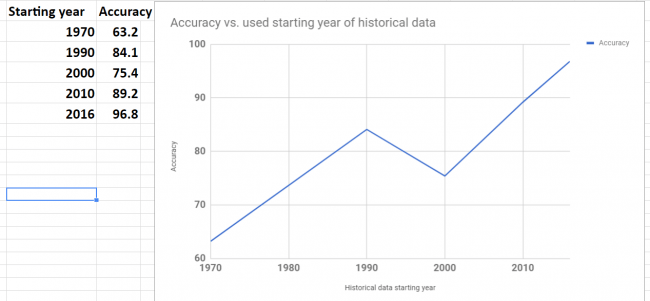prediction data stock prices with Prophet – accuracy vs used data range

Conclusion
We did not get good results (except one stock) in our experiments but we got the knowledge about possible range and distribution of error over the different stocks and time horizon (up to 20 days). Also it looks promising to try do forecast with different historical data range to check how it will affect performance. It would be interesting to see if the best accuracy that we got for one stock can be achieved for other stocks too.

I hope you enjoyed this post about using Prophet for prediction data stock prices. If you have any tips or anything else to add, please leave a comment in the reply box below.

Here is the script for stock data forecasting with python using Prophet.

import pandas as pd
from fbprophet import Prophet

steps_ahead = 20
fname_path="C:\\Users\\stock data folder"

fnames=['fn1.csv','fn2.csv', 'fn3.csv', 'fn4.csv', 'fn5.csv']
# output fields: actual, predicted, error, error in %, date
fields_number = 6

results= [[[0 for i in range(len(fnames))] for j in range(steps_ahead)] for k in range(fields_number)]

for ind in range(5):

fname=fname_path + "\\" + fnames[ind]

data = pd.read_csv (fname)

#keep only date and close
#delete Open, High,	Low  , Adj CLose, Volume
data.drop(data.columns[[1, 2, 3,5,6]], axis=1)
data.columns = ['ds', 'y', "", "", "", "", ""]

data_test = data[-steps_ahead:]
print (data_test)
data = data[:-steps_ahead]
print (data)

model = Prophet() #instantiate Prophet
model.fit(data);
future_stock_data = model.make_future_dataframe(periods=steps_ahead, freq = 'd')
forecast_data = model.predict(future_stock_data)
print (forecast_data[['ds', 'yhat', 'yhat_lower', 'yhat_upper']].tail(12))

step_count=0
for index, row in data_test.iterrows():

results[ind][step_count] = row['y']
results[ind][step_count] = row['ds']
step_count=step_count + 1

count_index = 0
for index, row in forecast_data.iterrows():
if count_index >= len(data)  :

step_count= count_index - len(data)
results[ind][step_count] = row['yhat']
results[ind][step_count] = results[ind][step_count] -  results[ind][step_count]
results[ind][step_count] = 100 * results[ind][step_count] / results[ind][step_count]

count_index=count_index + 1

for z in range (5):
for i in range (steps_ahead):
temp=""
for j in range (5):
temp=temp + " " + str(results[z][i][j])
print (temp)

print (z)


## Regression and Classification Decision Trees – Building with Python and Running Online

According to survey  Decision Trees constitute one of the 10 most popular data mining algorithms.
Decision trees used in data mining are of two main types:
Classification tree analysis is when the predicted outcome is the class to which the data belongs.
Regression tree analysis is when the predicted outcome can be considered a real number (e.g. the price of a house, or a patient’s length of stay in a hospital).

In the previous posts I already covered how to create Regression Decision Trees with python:

In this post you will find more simplified python code for classification and regression decision trees. Online link to run decision tree also will be provided. This is very useful if you want see results immediately without coding.

To run the code provided here you need just change file path to file containing data. The Decision Trees in this post are tested on simple artificial dataset that was motivated by doing feature selection for blog data:

Getting Data-Driven Insights from Blog Data Analysis with Feature Selection

Dataset
Our dataset consists of 3 columns in csv file and shown below. It has 2 independent variables (features or X columns) – categorical and numerical, and dependent numerical variable (target or Y column). The script is assuming that the target column is the last column. Below is the dataset that is used in this post:


X1	X2	Y
red	1	100
red	2	99
red	1	85
red	2	100
red	1	79
red	2	100
red	1	100
red	1	85
red	2	100
red	1	79
blue	2	22
blue	1	20
blue	2	21
blue	1	13
blue	2	10
blue	1	22
blue	2	20
blue	1	21
blue	2	13
blue	1	10
blue	1	22
blue	2	20
blue	1	21
blue	2	13
blue	1	10
blue	2	22
blue	1	20
blue	2	21
blue	1	13
green	2	10
green	1	22
green	2	20
green	1	21
green	2	13
green	1	10
green	2	22
green	1	20
green	1	13
green	2	22
green	1	20
green	2	21
green	1	13
green	2	10


You can use dataset with different number of columns for independent variables without changing the code.

For converting categorical variable to numerical we use here pd.get_dummies(dataframe) method from pandas library. Here dataframe is our input data. So the column with “green”, “red”, “yellow” will be transformed in 3 columns with 0,1 values in each (one hot encoding scheme). Below are the few first rows after converting:


N   X2  X1_blue  X1_green  X1_red    Y
0    1      0.0       0.0     1.0  100
1    2      0.0       0.0     1.0   99
2    1      0.0       0.0     1.0   85
3    2      0.0       0.0     1.0  100


Python Code
Two scripts are provided here – regressor and classifier. For classifier the target variable should be categorical. We use however same dataset but convert numerical continuous variable to classes with labels (A,B,C) within the script based on inputted bin ranges ([15,50,100] which means bins 0-15, 15.001-50, 50.001-100). We use this after applying get_dummies

What if you have categorical target? Calling get_dummies will convert it to numerical too but we do not want this. In this case you need specify explicitly what columns need to be converted via parameter columns As per the documentation:
columns : list-like, default None. This is column names in the DataFrame to be encoded. If columns is None then all the columns with object or category dtype will be converted. 
In our example we would need to do specify column X1 like this:
dataframe=pd.get_dummies(dataframe, columns=[“X1”])

The results of running scripts are decision trees shown below:
Decision Tree RegressionDecision Tree Classification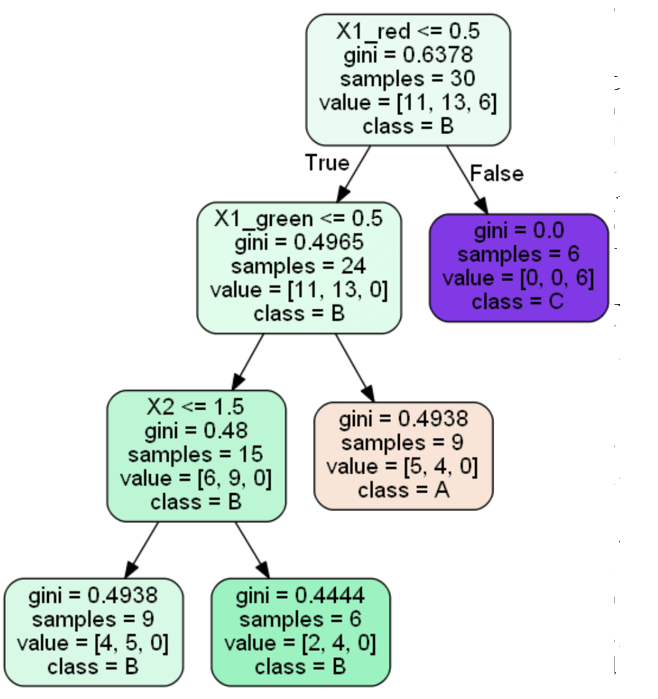Running Decision Trees Online
In case you do not want to play with python code, you can run Decision Tree algorithms online at ML Sandbox
All that you need is just enter data into the data fields, here are the instructions:

1. Go to ML Sandbox
2. Select Decision Classifier OR Decision Regressor
3. Enter data (first row should have headers) OR click “Load Default Values” to load the example data from this post. See screenshot below
4. Click “Run Now“.
5. Click “View Run Results
6. If you do not see yet data wait for a minute or so and click “Refresh Page” and you will see results
7. Note: your dependent variable (target variable or Y variable) should be in most right column. Also do not use space in the words (header and data)

Conclusion
Decision Trees belong to the top 10 machine learning or data mining algorithms and in this post we looked how to build Decision Trees with python. The source code provided is the end of this post. We looked also how do this if one or more columns are categorical. The source code was tested on simple categorical and numerical example and provided in this post. Alternatively you can run same algorithm online at ML Sandbox

References

Here is the python computer code of the scripts.
DecisionTreeRegressor


# -*- coding: utf-8 -*python computer code-

import pandas as pd
from sklearn.cross_validation import train_test_split
from sklearn.tree import DecisionTreeRegressor

import subprocess

from sklearn.tree import  export_graphviz

def visualize_tree(tree, feature_names):

with open("dt.dot", 'w') as f:

export_graphviz(tree, out_file=f, feature_names=feature_names,  filled=True, rounded=True )

command = ["C:\\Program Files (x86)\\Graphviz2.38\\bin\\dot.exe", "-Tpng", "C:\\Users\\Owner\\Desktop\\A\\Python_2016_A\\dt.dot", "-o", "dt.png"]

try:
subprocess.check_call(command)
except:
exit("Could not run dot, ie graphviz, to "
"produce visualization")

filename = "C:\\Users\\Owner\\Desktop\\A\\Blog Analytics\\data1.csv"
dataframe = pd.read_csv(filename, sep= ',' )

cols = dataframe.columns.tolist()
last_col_header = cols[-1]

dataframe=pd.get_dummies(dataframe)
cols = dataframe.columns.tolist()

cols.insert(len(dataframe.columns)-1, cols.pop(cols.index(last_col_header)))
dataframe = dataframe.reindex(columns= cols)

print (dataframe)

array = dataframe.values
X = array[:,0:len(dataframe.columns)-1]
Y = array[:,len(dataframe.columns)-1]
print ("--X----")
print (X)
print ("--Y----")
print (Y)

X_train, X_test, y_train, y_test = train_test_split( X, Y, test_size = 0.3, random_state = 100)

clf = DecisionTreeRegressor( random_state = 100,
max_depth=3, min_samples_leaf=4)
clf.fit(X_train, y_train)

visualize_tree(clf, dataframe.columns)


DecisionTreeClassifier


# -*- coding: utf-8 -*-

import pandas as pd
from sklearn.cross_validation import train_test_split
from sklearn.tree import DecisionTreeClassifier

import subprocess

from sklearn.tree import  export_graphviz

def visualize_tree(tree, feature_names, class_names):

with open("dt.dot", 'w') as f:

export_graphviz(tree, out_file=f, feature_names=feature_names,  filled=True, rounded=True, class_names=class_names )

command = ["C:\\Program Files (x86)\\Graphviz2.38\\bin\\dot.exe", "-Tpng", "C:\\Users\\Owner\\Desktop\\A\\Python_2016_A\\dt.dot", "-o", "dt.png"]

try:
subprocess.check_call(command)
except:
exit("Could not run dot, ie graphviz, to "
"produce visualization")

values=[15,50,100]
def convert_to_label (a):
count=0
for v in values:
if (a <= v) :
return chr(ord('A') + count)
else:
count=count+1

filename = "C:\\Users\\Owner\\Desktop\\A\\Blog Analytics\\data1.csv"
dataframe = pd.read_csv(filename, sep= ',' )

cols = dataframe.columns.tolist()
last_col_header = cols[-1]
dataframe=pd.get_dummies(dataframe)
cols = dataframe.columns.tolist()

print (dataframe)

for index, row in dataframe.iterrows():
dataframe.loc[index, "Y"] = convert_to_label(dataframe.loc[index, "Y"])

cols.insert(len(dataframe.columns)-1, cols.pop(cols.index('Y')))
dataframe = dataframe.reindex(columns= cols)

print (dataframe)

array = dataframe.values
X = array[:,0:len(dataframe.columns)-1]
Y = array[:,len(dataframe.columns)-1]
print ("--X----")
print (X)
print ("--Y----")
print (Y)

X_train, X_test, y_train, y_test = train_test_split( X, Y, test_size = 0.3, random_state = 100)

clf = DecisionTreeClassifier(criterion = "gini", random_state = 100,
max_depth=3, min_samples_leaf=4)

clf.fit(X_train, y_train)

clm=dataframe[last_col_header]
clmvalues = clm.unique()
visualize_tree(clf, dataframe.columns, clmvalues )